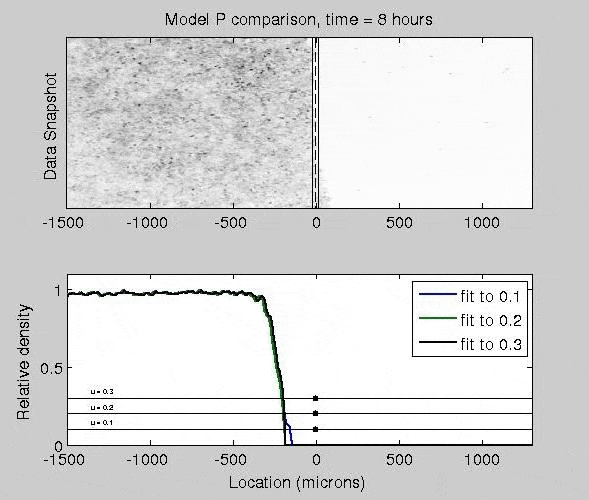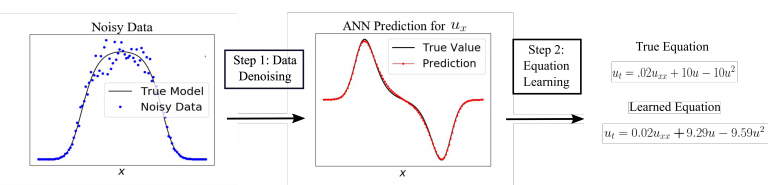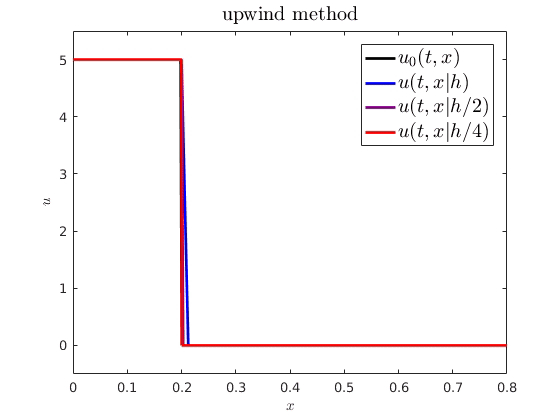# Research

You can read my research statement here.

I aim to use mathematical models to answer biological questions. This often requires mathematical models to describe how various phenomena occur in combination with analysis to describe the overall patterns we can expect.  While my main expertise is in partial differential equation (PDEs) models in biology, I also have significant experience with ordinary differential equations, inverse problems, and statistical methods.

### Mathematical Models of Epidermal Wound HealingVarious mechanical and biochemical processes regulate how keratinocyte cells from our epithelial skin layer migrate into a wound. For example, these cells maintain cell-cell adhesions, or physical connections to their neighbors, as they migrate. I have used PDE models to describe how cells from experiments use physical connections to pull their followers into the wound with them. We validated this model hypothesis by re-doing this experiment with cell populations with decreased cell-cell adhesion levels and indeed saw weakened migration rates into the wound.

The mitogen-activated protein kinase (MAPK) pathway strongly controls how epithelial cells heal a wound, as activated cells exhibit fast migration rates and deactivated cells tend to proliferate. To quantify how different patterns of biochemical activation regulate how epithelial cells close a wound, I derived a biochemically-structured Fisher Kolmogorov equation. Analysis of this equation lead to a self-similar traveling wave solution, and time-dependent nonlinear PDE models. This framework broadly applicable to many reaction-diffusion and chemotaxis equations.

J. Nardini, D. Chapnick, X. Liu, D. M. Bortz. Modeling keratinocyte wound healing dynamics: cell-cell adhesion promotes sustained collective migration. J. Theor. Biol., 7 July 2016, 103-117. DOI: 10.1016/j.jtbi.2016.04.015.

J. Nardini, D. M. Bortz. Investigation of a Structured Fisher’s Equation with Applications in Biochemistry. SIAM J. Appl. Math. Vol. 78, No. 3: pp. 1712-1736. DOI: 10.1137/16M1108546.

### Inverse Problems in Biology

Given an experimental dataset, we are often interested in selecting a mathematical model to describe the data and estimating parameters describing the effects of certain processes on the resulting data.

Choosing a mathematical model to accurately describe a noisy data set is a challenging process. Equation learning is a recent field of research that uses concepts from machine learning to infer a mathematical model directly from noisy data. Even small noise levels (such as 5%) can corrupt derivative computations which in turn leads to inaccurate PDE model recovery from data. Our recent study combined an artificial neural network (ANN) with the PDE-FIND algorithm to accurately select PDE models from data with up to 25% noise levels. We believe this will lead these methods to soon be used to infer models from real biological data.When comparing spatiotemporal processes to mathematical models, we often need numerical approximations to simulate the model. For example, on the right, I have plotted an analytical PDE solution (black) against some numerical  approximations (getting more accurate from blue to red). An interesting question is how numerical error from these approximations influences parameter estimation and uncertainty quantification. I showed how we can expect a numerical cost function and parameter estimator to behave when using standard finite difference schemes to approximate advection-driven PDE models. I also derived an autocorrelative statistical model to account for numerical diffusion from first order methods and improve parameter estimation.

J. Lagergren, J. Nardini, G. M. Lavigne, E. M. Rutter, K. B. Flores, Learning partial differential equations for biological transport models from noisy spatiotemporal data. arXiv.1902.04733. Accepted for Proceedings of the Royal Society A.

J. Nardini, D. M. Bortz. The Influence of Numerical Error on an Inverse Problem Methodology in PDE Models. Inverse Problems 35 (6) 065003, 2019DOI: 10.1088/1361-6420/ab10bb

K. Adoteye, R. Baraldi, K. Flores, J. Nardini, H. T. Banks, W. C. Thompson. Correlation of parameter estimators for models admitting multiple parameterizations. Int. J. Pure Appl. Math., 105(3) 497, 2015. DOI: 10.12732/ijpam.v105i3.16.

T. Huffman, K. Link, J. Nardini, L. Poag, K. Flores, H.T. Banks, B. Biasco, J. Jungfleisch, J. Diez. A mathematical model of RNA3 recruitment in the replication cycle of brome mosaic virus. Int. J. Pure Appl. Math., 92(1) 27, 2014. DOI: 10.12732/ijpam.v92i1.3.

H.T. Banks, A. Choi, T. Huffman, J. Nardini, L. Poag, W.C. Thompson. Quantifying CFSE label decay in flow cytometry data. Appl. Math. Lett., 26(5) 571, 2013. DOI: 10.1016/j.aml.2012.12.010.